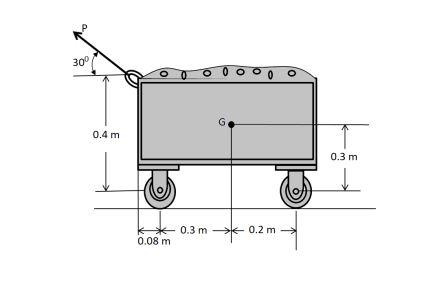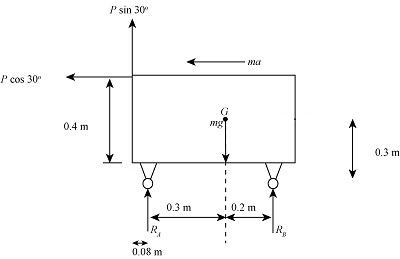# A force of P= 300 N is applied to the 60 kg cart determine the reactions at both the wheels at A...

## Question:

A force of P= 300 N is applied to the 60 kg cart determine the reactions at both the wheels at A and both the wheels at B, Also. what is the acceleration of the cart? The mass center of the cart is at G.## Equilibrium of a body:

A body stays in mechanical equilibrium if the forces applied on it do not produce any net effect on the body or the applied forces in a particular direction do not change the equilibrium position of the body in that direction.

## Answer and Explanation:

Given Data

• The mass of the cart is: {eq}m = 60\;{\rm{kg}} {/eq}
• The force applied is: {eq}P = 300\;{\rm{N}} {/eq}
• The acceleration due to gravity is: {eq}g = 9.81\;{\rm{m/}}{{\rm{s}}^{\rm{2}}} {/eq}

The figure below represents the free body diagram of the cart.(c)

The equilibrium of forces in horizontal direction is,

{eq}P\cos 30^\circ = ma {/eq}

Here, the acceleration of car is {eq}a. {/eq}

Substitute the values in above equation.

{eq}\begin{align*} 300\;{\rm{N}} \times \dfrac{{\sqrt 3 }}{2}& = 60\;{\rm{kg}} \times a\\ a& = \dfrac{{259.807\;{\rm{kg}} \cdot {\rm{m/}}{{\rm{s}}^{\rm{2}}}}}{{60\;{\rm{kg}}}}\\ a& = 4.33\;{\rm{m/}}{{\rm{s}}^{\rm{2}}} \end{align*} {/eq}

(b)

The moment equilibrium about point A is,

{eq}P\cos 30^\circ \times 0.4 + {R_B} \times 0.5 = ma \times 0.3 + P\sin 30^\circ \times 0.08 + mg \times 0.3 {/eq}

Substitute the values in above equation.

{eq}\begin{align*} 300\;{\rm{N}} \times \dfrac{{\sqrt 3 }}{2} \times 0.4\;{\rm{m}} - 300\;{\rm{N}} \times 0.5 \times 0.08\;{\rm{m}} + {R_B} \times 0.5\;{\rm{m}}& = 60\;{\rm{kg}}\left( {4.33\;{\rm{m/}}{{\rm{s}}^{\rm{2}}}} \right) \times 0.3\;{\rm{m}} + 60\;{\rm{kg}}\left( {9.81\;{\rm{m/}}{{\rm{s}}^{\rm{2}}}} \right) \times 0.3\;{\rm{m}}\\ {R_B}& = \dfrac{{162.59\;{\rm{N}} \cdot {\rm{m}}}}{{0.5\;{\rm{m}}}}\\ {R_B}& = 325.193\;{\rm{N}} \end{align*} {/eq}

Thus, the reaction force at B is {eq}{R_B} = 325.193\;{\rm{N}} {/eq}

(c)

The equilibrium of forces in vertical direction is,

{eq}{R_A} + {R_B} + P\sin 30^\circ = mg {/eq}

Substitute the values in above equation.

{eq}\begin{align*} {R_A} + 325.193\;{\rm{N}} + 300\;{\rm{N}} \times 0.5& = 60\;{\rm{kg}} \times 9.81\;{\rm{m/}}{{\rm{s}}^{\rm{2}}}\\ {R_A}& = 588.6\;{\rm{N}} - 475.193\;{\rm{N}}\\ {R_A}& = 113.47\;{\rm{N}} \end{align*} {/eq}

Thus, the reaction force at A is {eq}{R_A} = 113.407\;{\rm{N}} {/eq}